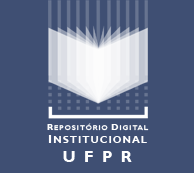# Biblioteca Digitalde Periódicosda Universidade Federal do Paraná

## Open Journal Systems

### INTEGRATION OF A MATHEMATICAL EXERGOECONOMIC OPTIMIZATION PROCEDURE WITH A PROCESS SIMULATOR: APPLICATION TO THE CGAM SYSTEM

L. S. R. Vieira, J. L. M. Donatelli, M. E. C. Cruz

#### Abstract

In the present work a recent development and implementation of an integrated approach for mathematical exergoeconomic optimization of thermal systems is described. The proposed integrated approach is designed to exploit the computational power of a professional process simulator, so that the optimization procedure may disregard the variables associated with the thermodynamic balance equations, and thus deal with the decision variables only. To demonstrate its capabilities, the integrated approach is here applied to the CGAM system. Complete simulation of the CGAM system requires more than 200 variables. In a conventional mathematical optimization procedure, all variables must be handled simultaneously. In the proposed integrated exergoeconomic optimization approach, only 5 decision variables are manipulated by the optimization kernel, while the remaining variables are managed by the simulator. Because the thermodynamic balance equations are decoupled from the optimization problem, the proposed approach is computationally efficient, and can therefore be applied to optimize complex thermal systems.

#### Keywords

Mathematical optimization; exergoeconomic optimization; thermal systems; process simulator; exergy; CGAM

#### Full Text:

PDF

DOI: http://dx.doi.org/10.5380/reterm.v4i2.5413